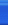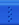# 分享知识C++博客首页新随笔联系聚合管理1）看看两个语句：
typedef struct _person
{
char m_strName;
int m_nAge;
}*TPPerson;

#define DPPerson \
struct _person \
{ \
char m_strName;\
int m_nAge; \
}*

tp1 = tp2; dp1 = dp2;

2）define SQUARE(X) (X)*(X)

3）字符串定义和标志粘贴

#define TRACE(X) cout<< #X << " = " << X << endl;
#define MEMBER(A) char* A##_string;int A##_size

#include
#define TRACE(X) cout<< #X << " = " << X << endl;
#define MEMBER(A) char* A##_string;int A##_size
using namespace std;

int main()
{
for(int i = 0;i < 10;i++)
TRACE((3+i));
MEMBER(one);
one_string = "Hello";
one_size = 4;
cout << one_string << ":" << one_size << endl;

return 0;
}

(3+i) = 3
(3+i) = 4
(3+i) = 5
(3+i) = 6
(3+i) = 7
(3+i) = 8
(3+i) = 9
(3+i) = 10
(3+i) = 11
(3+i) = 12
Hello:4

posted on 2006-04-12 15:57 史传红 阅读(382) 评论(1)  编辑 收藏 引用 所属分类: C/C++细节知识### Feedback

# re: C++ Thinking 学习笔记之二----说说C/C++的预处理宏 2006-04-12 18:08 Stone Jiang

回复  更多评论